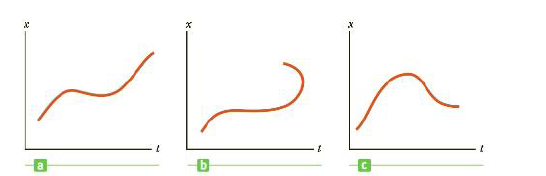Chapter 2.2, Problem 2.4QQ

Chapter
Section
Textbook Problem

The three graphs in Figure 2.13 represent the position vs. time for objects moving along the x-axis. Which, if any, of these graphs is not physically possible?Figure 2.13 (Quick Quiz 2.4) Which position vs. time curve is impossible?

To determine
The position versus time graph which is not physically possible.

Explanation

Given info: The figures show the position versus time curve of objects.

A particle can only be seen at one position at an instant.

Explanation:

The curve shown in option (b) has two different positions for the same object at the same instant...

Still sussing out bartleby?

Check out a sample textbook solution.

See a sample solution

The Solution to Your Study Problems

Bartleby provides explanations to thousands of textbook problems written by our experts, many with advanced degrees!

Get Started